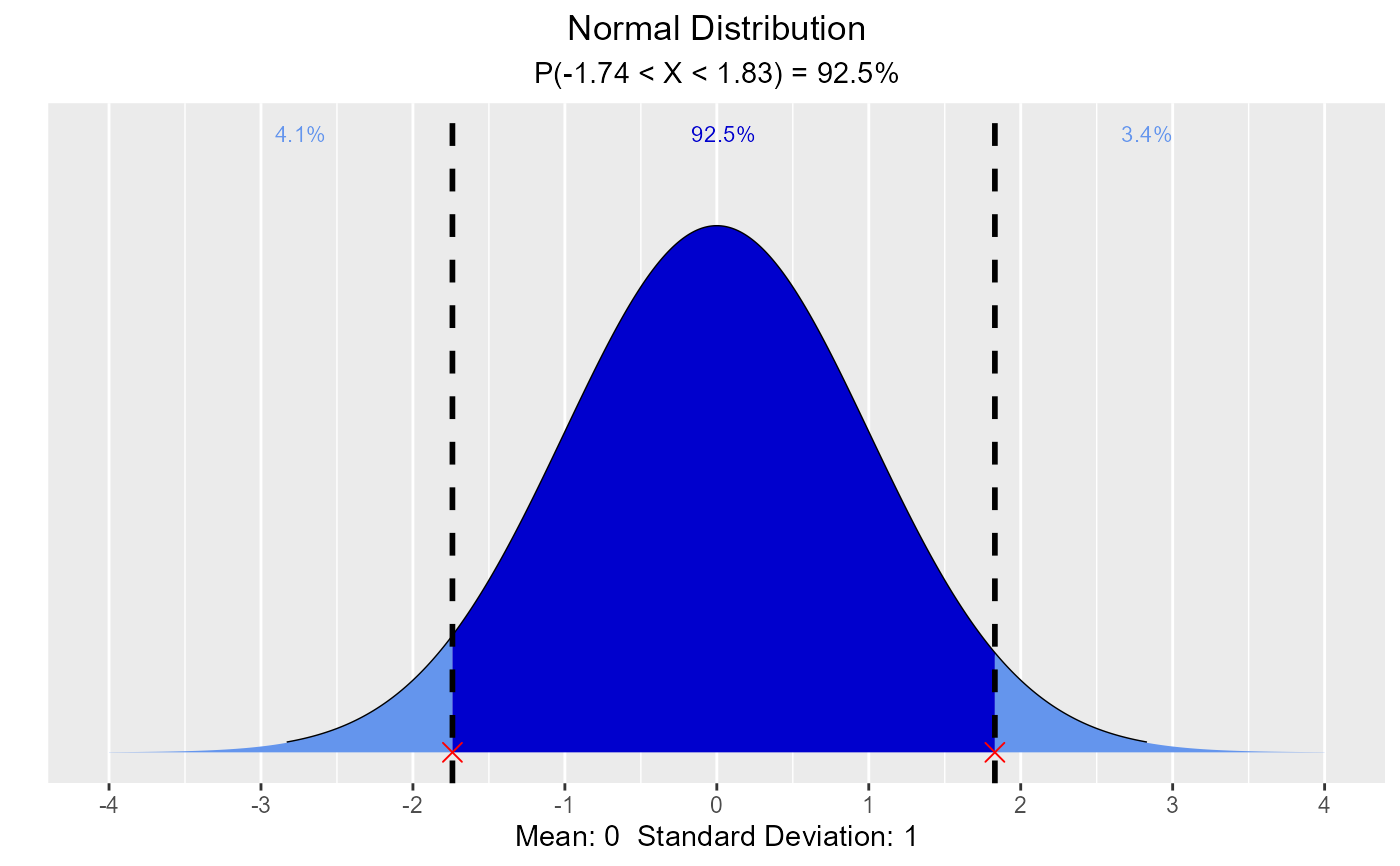Visualize how changes in mean and standard deviation affect the shape of the normal distribution. Compute & visualize quantiles out of given probability and probability from a given quantile.

vdist_normal_plot(mean = 0, sd = 1, print_plot = TRUE)

vdist_normal_perc(
probs = 0.95,
mean = 0,
sd = 1,
type = c("lower", "upper", "both"),
print_plot = TRUE
)

vdist_normal_prob(
perc = 3,
mean = 0,
sd = 1,
type = c("lower", "upper", "both"),
print_plot = TRUE
)

## Arguments

mean Mean of the normal distribution. Standard deviation of the normal distribution. logical; if TRUE, prints the plot else returns a plot object. Probability value. Lower tail, upper tail or both. Quantile value.

Normal

## Examples

# visualize normal distribution
vdist_normal_plot()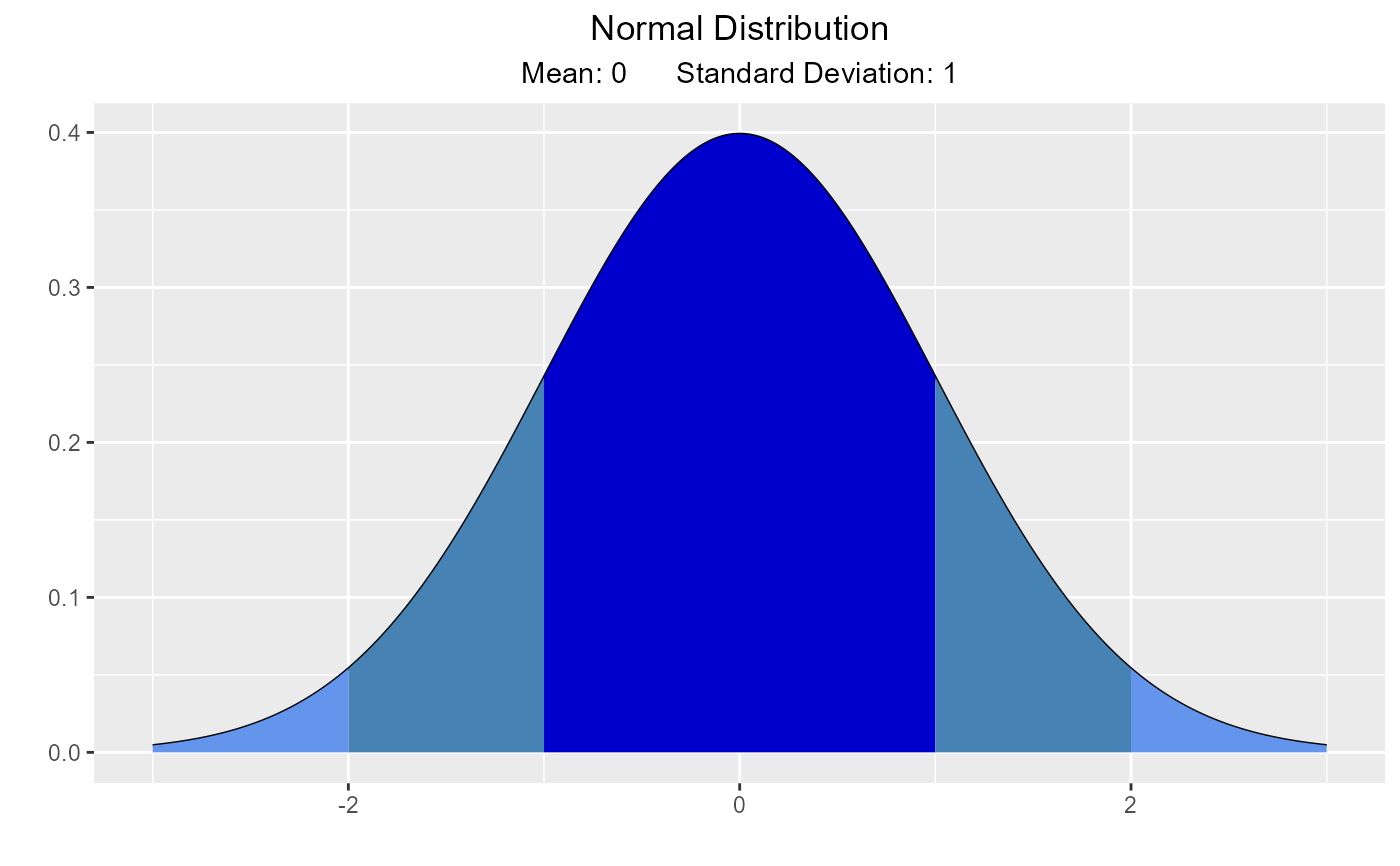vdist_normal_plot(mean = 2, sd = 0.6)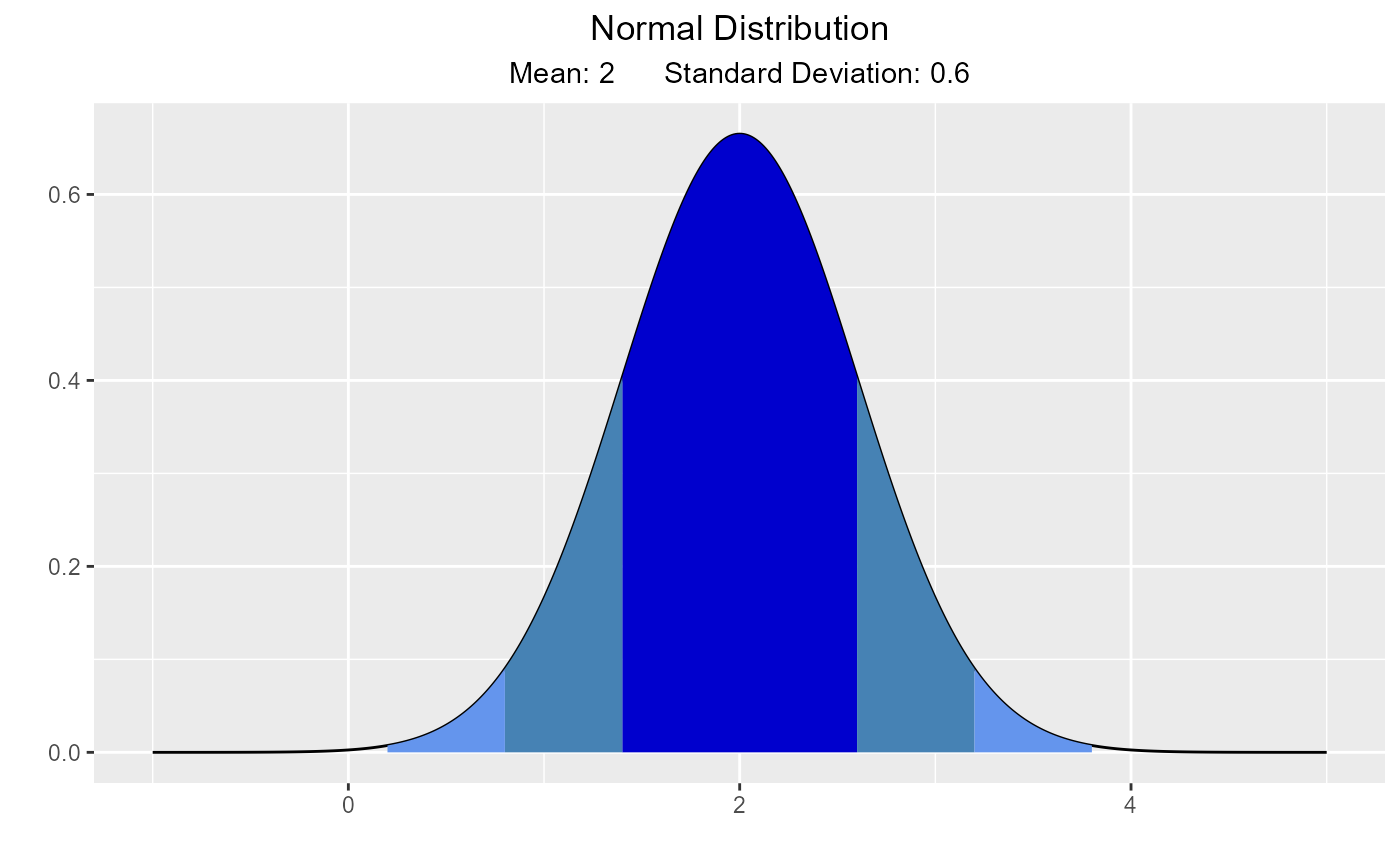# visualize quantiles out of given probability
vdist_normal_perc(0.95, mean = 2, sd = 1.36)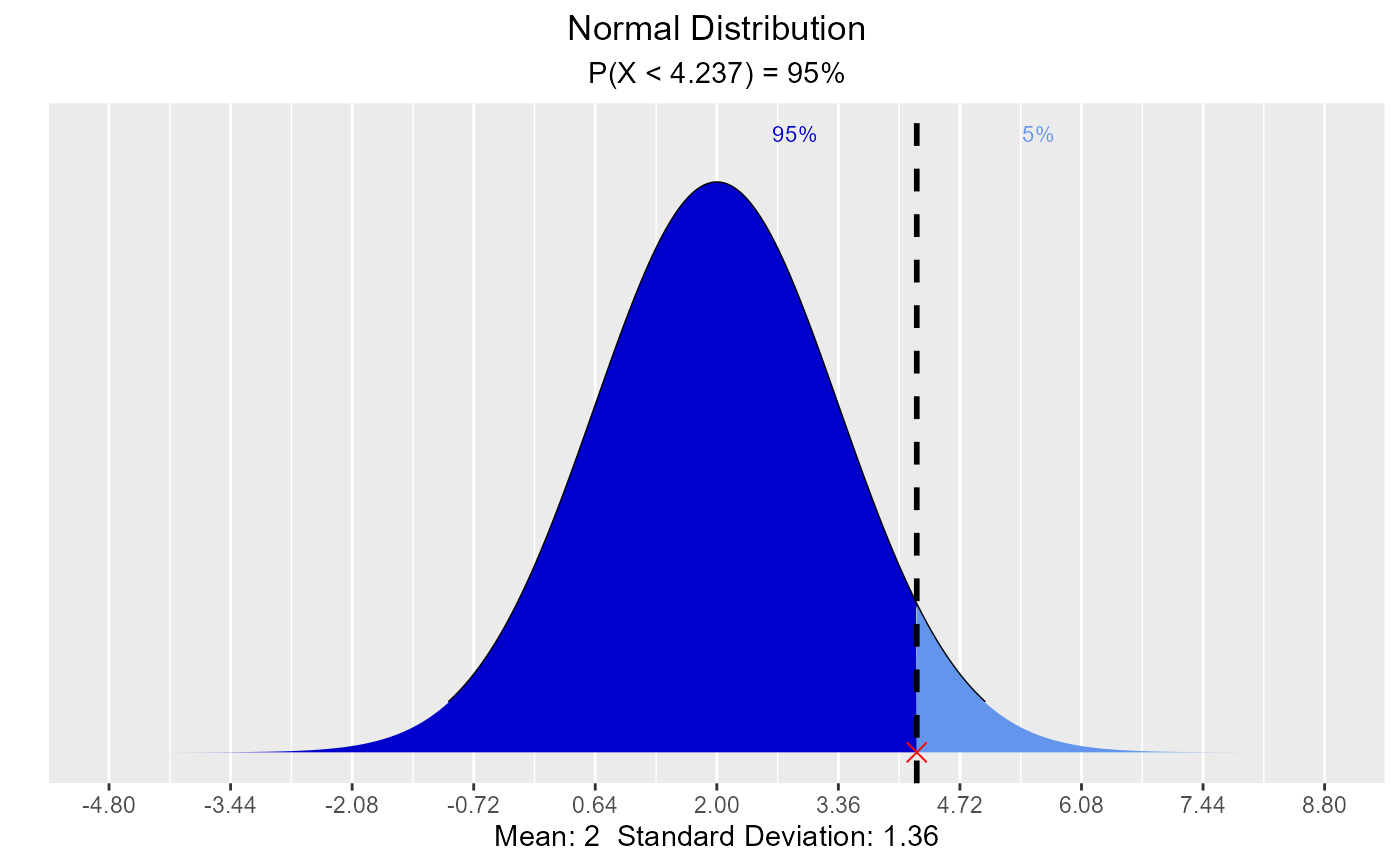vdist_normal_perc(0.3, mean = 2, sd = 1.36, type = 'upper')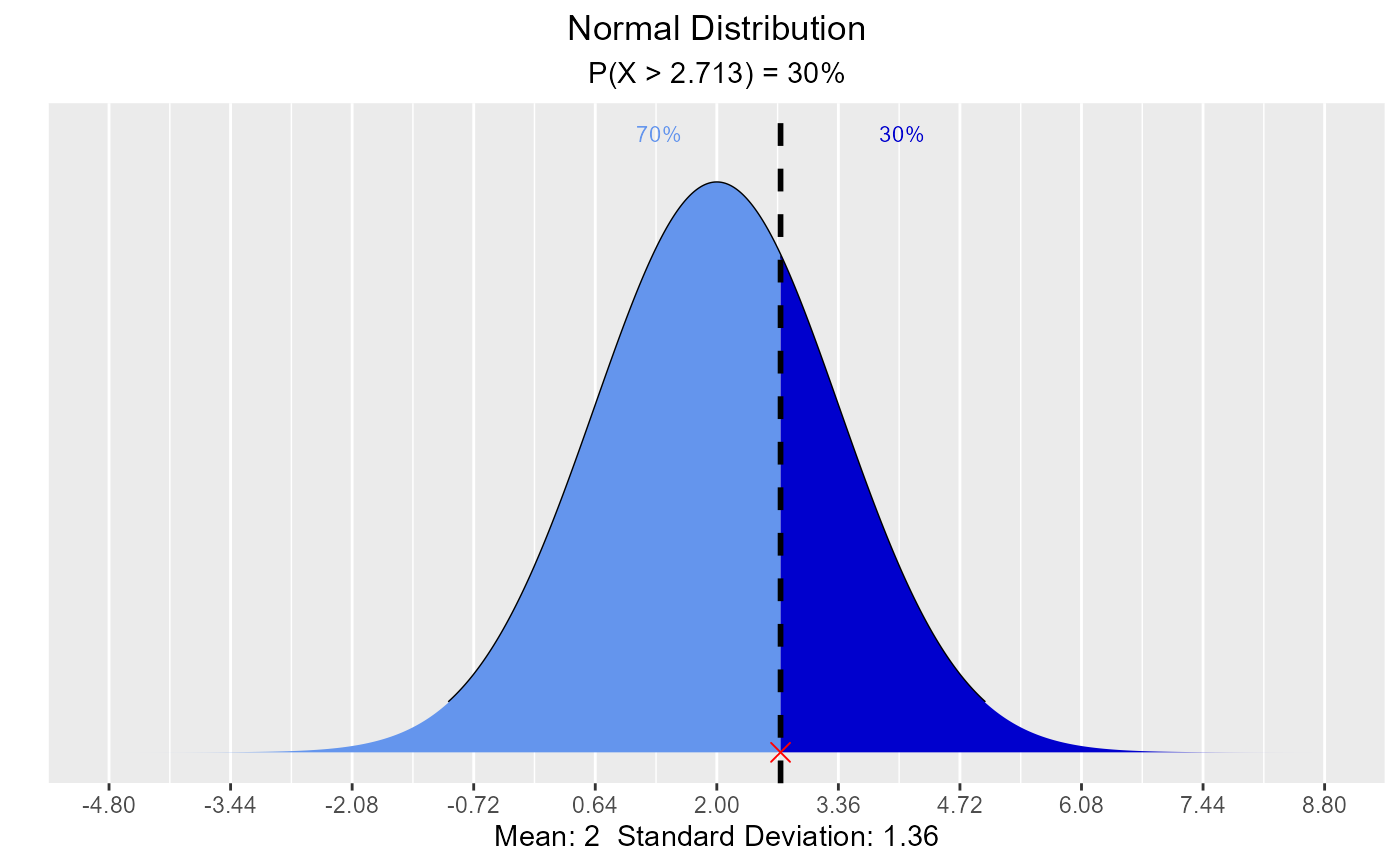vdist_normal_perc(0.95, mean = 2, sd = 1.36, type = 'both')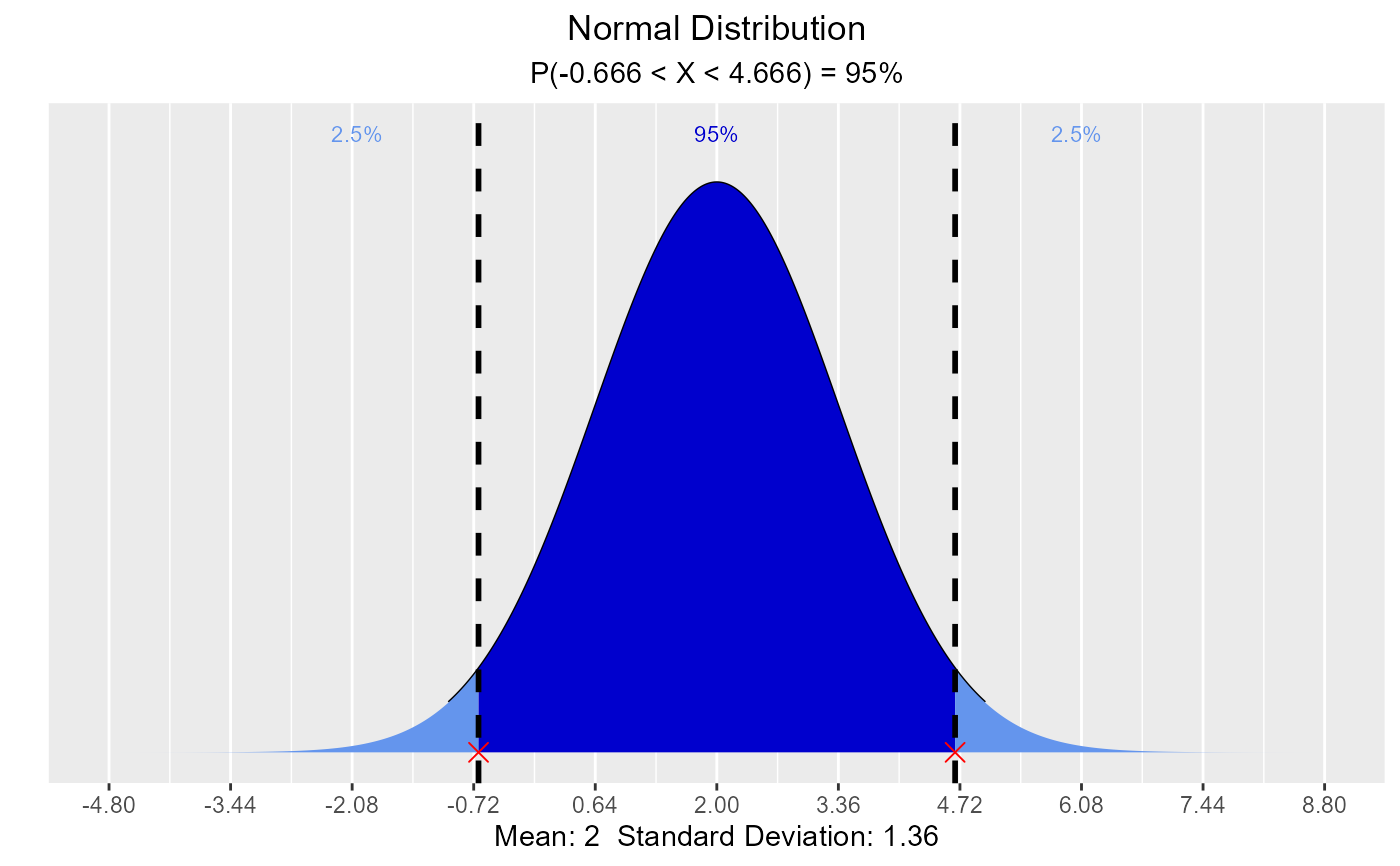# visualize probability from a given quantile
vdist_normal_prob(3.78, mean = 2, sd = 1.36)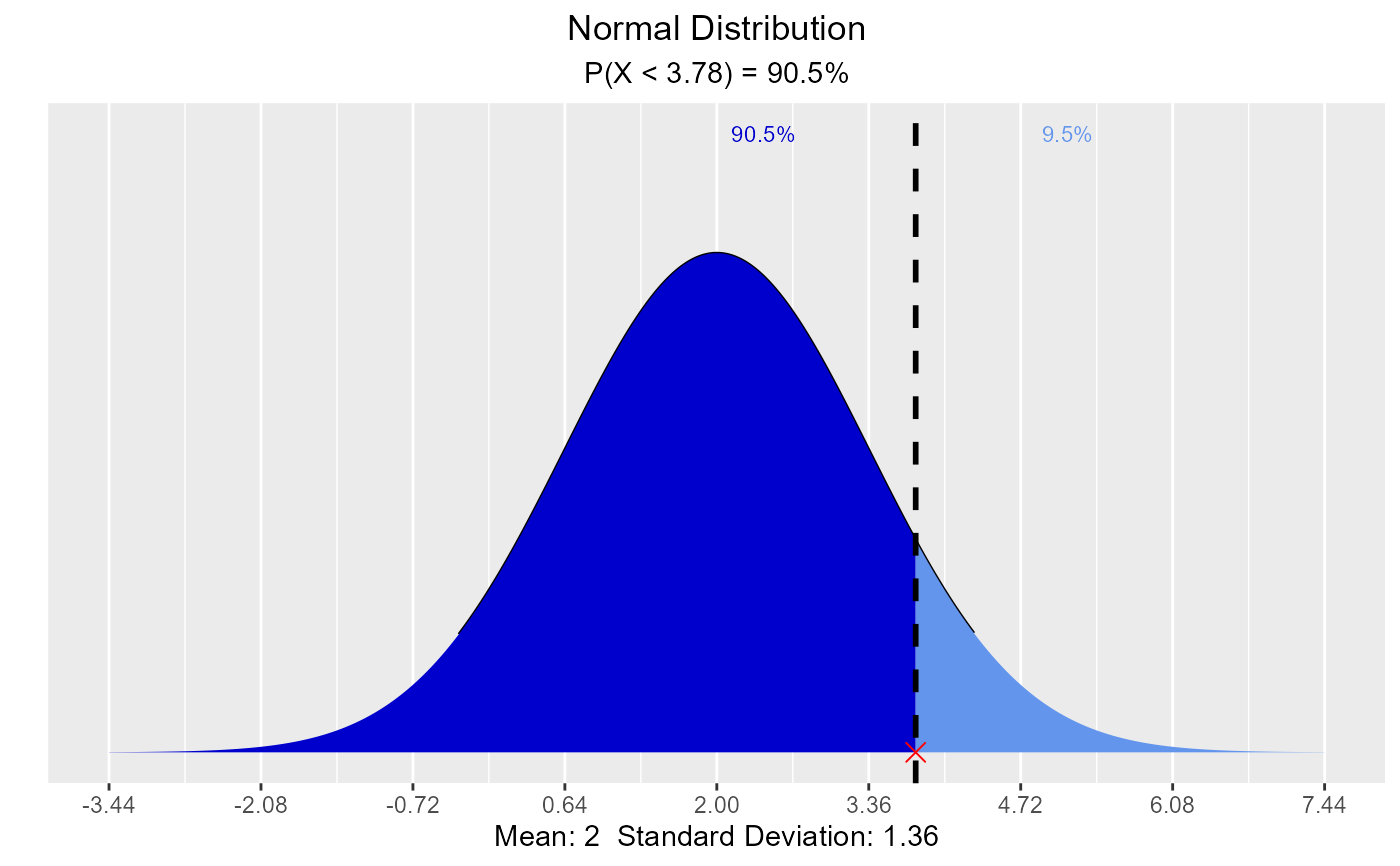vdist_normal_prob(3.43, mean = 2, sd = 1.36, type = 'upper')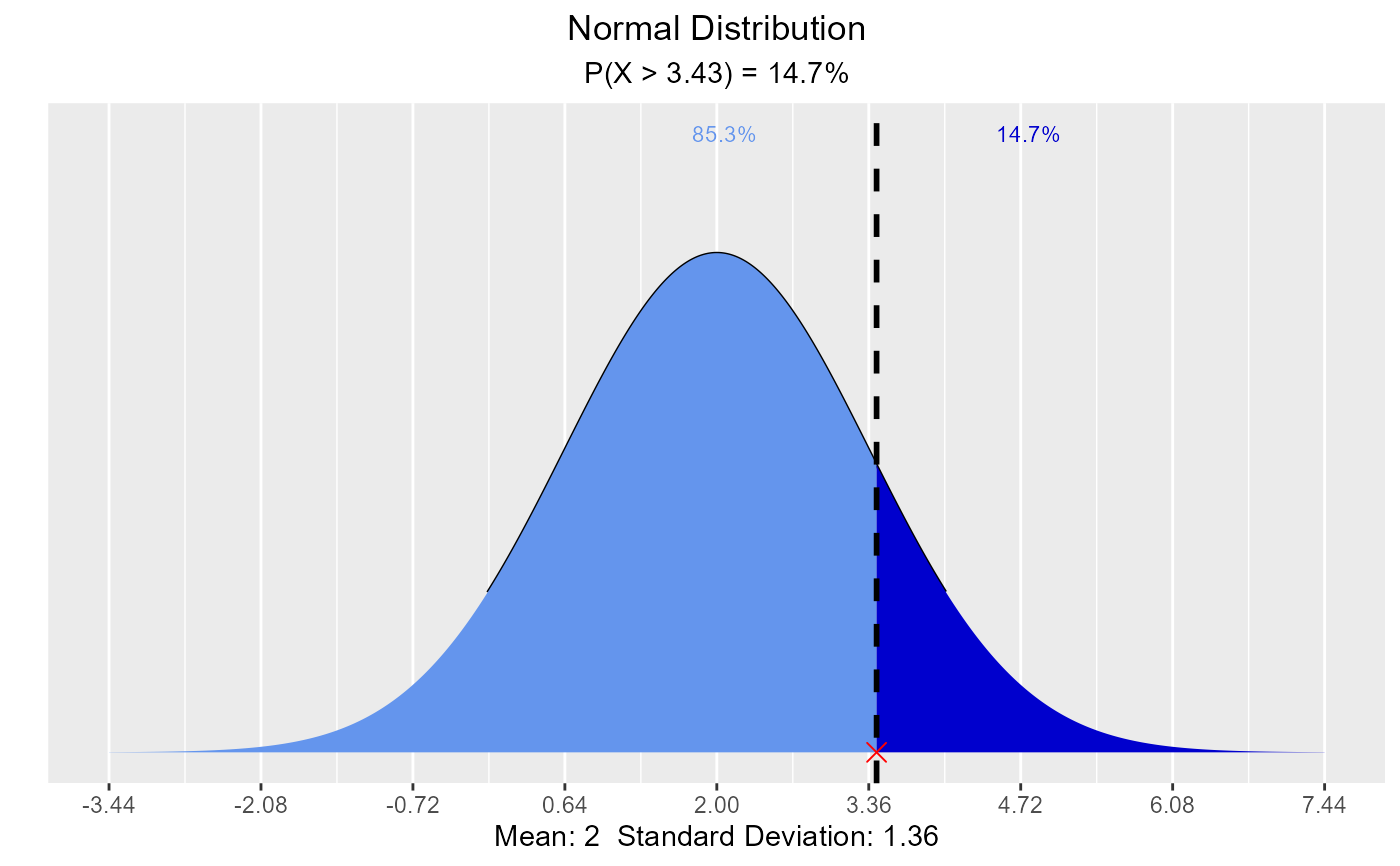vdist_normal_prob(c(-1.74, 1.83), type = 'both')